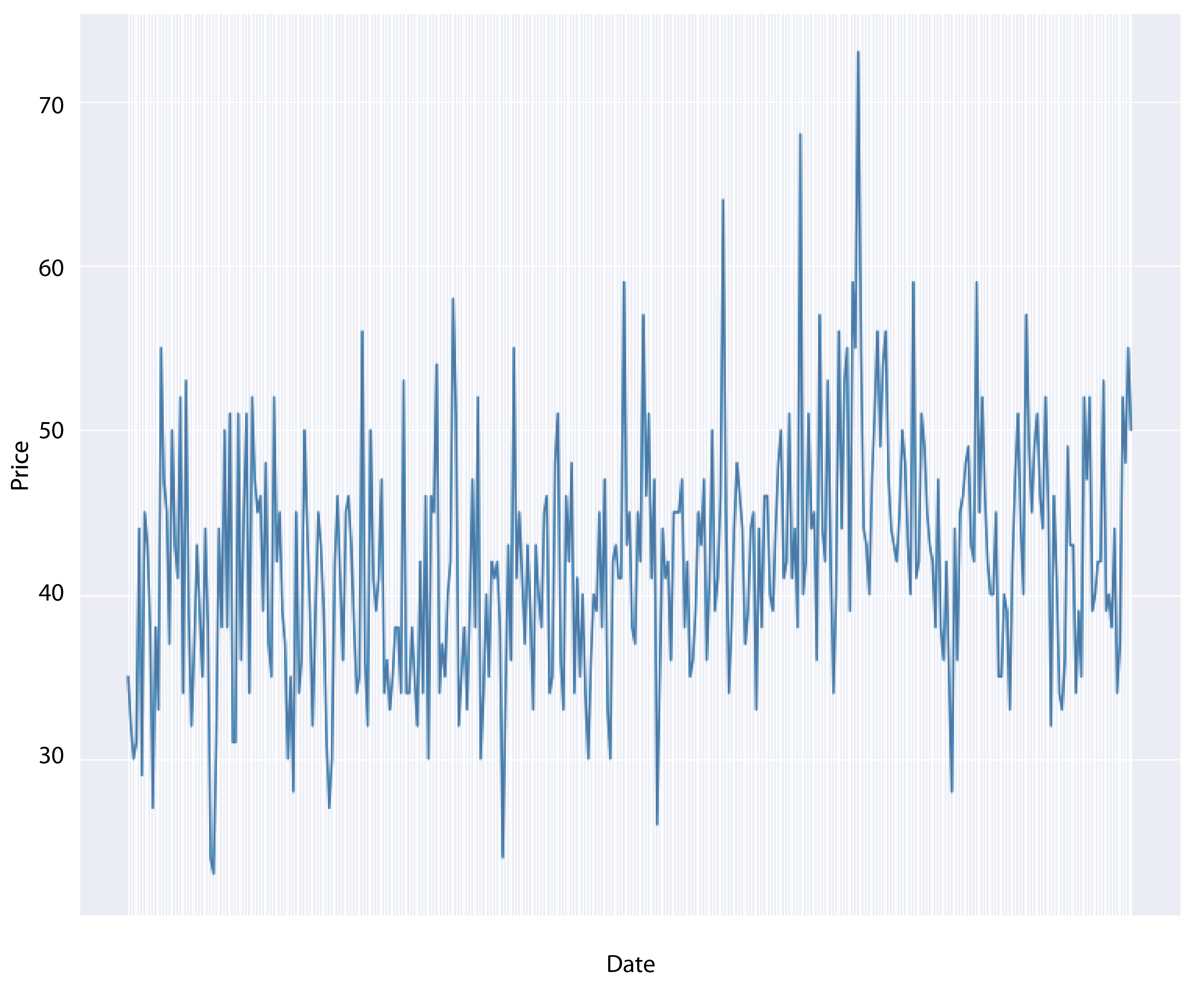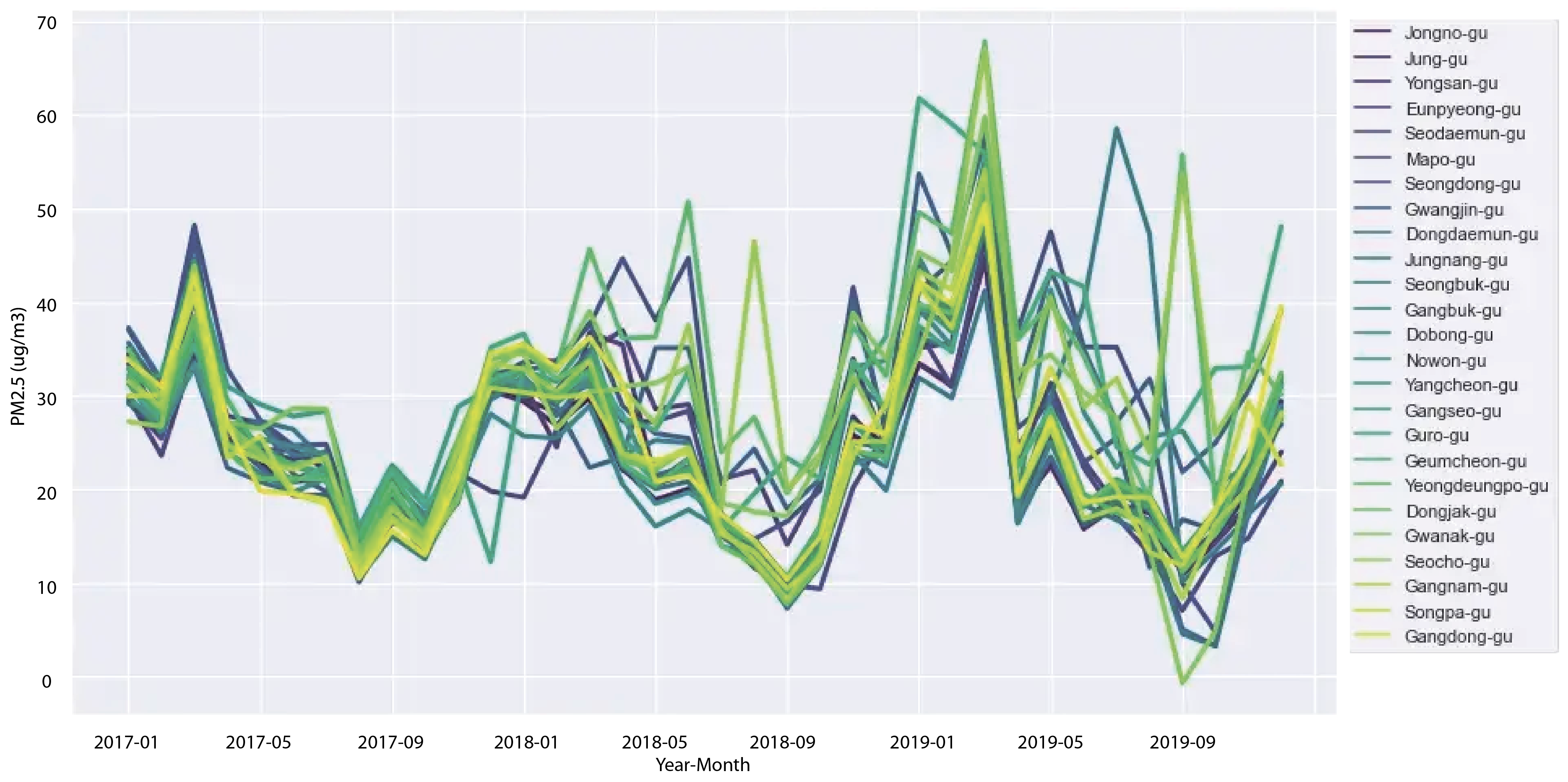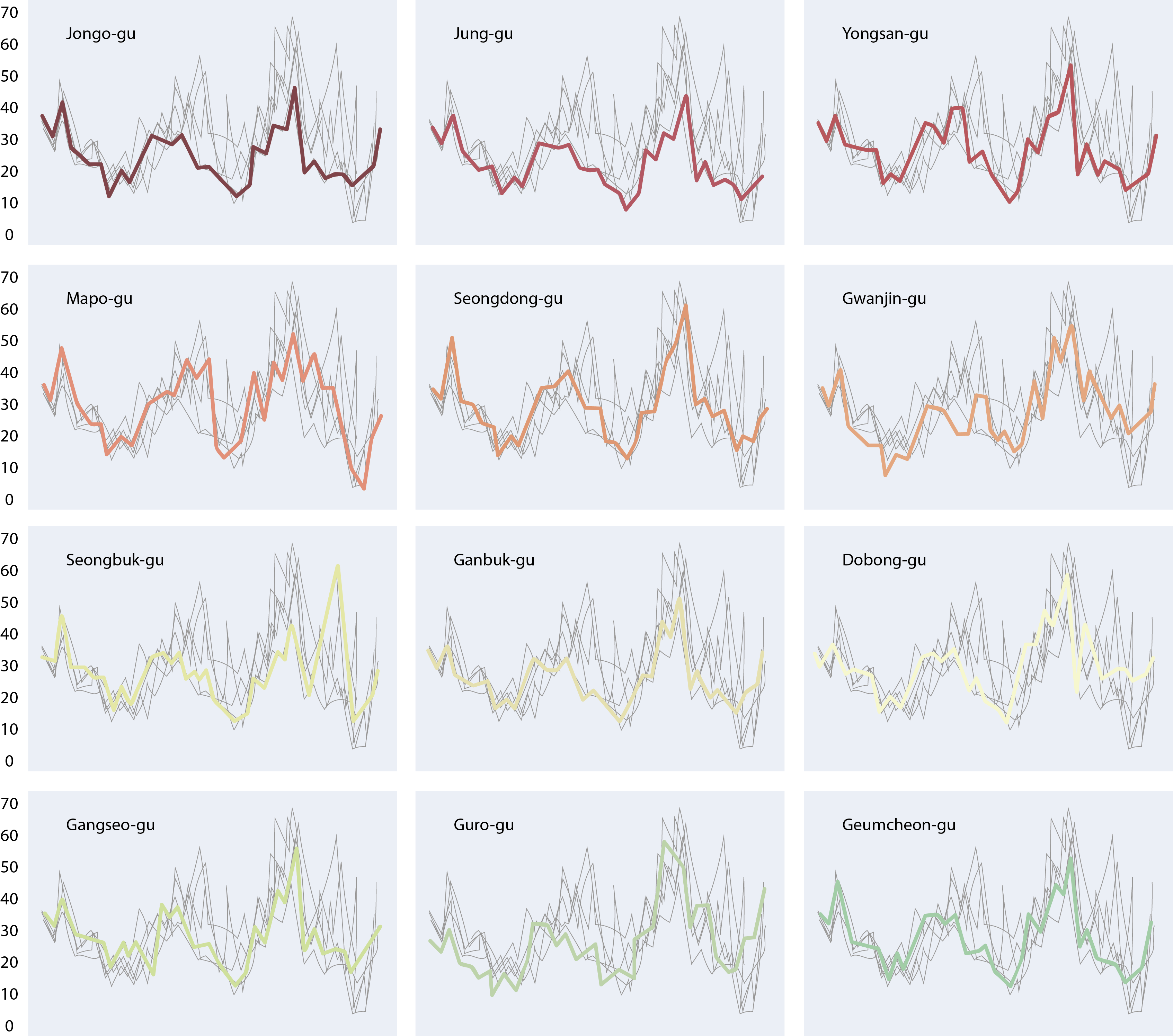Course Content

# Time Series Analysis

1. Time Series: Let's Start

2. Time Series Processing

3. Time Series Visualization

Time Series Analysis

##Visualization

In the 3rd section, you will get acquainted with the visualization of time series with only one dependent variable and multiple time series with many variables that must be visualized on the same plot.
Time series visualization is one of the most important stages of data processing, allowing the expert to evaluate the big picture and roughly understand the nature of the data (stationarity, seasonality, cyclicality, etc.)

The `matplotlib` library is used for visualization. An example of visualizing time series with one variable:### What problems can arise when rendering multiple time series on the same plot?

If the number of time series you want to visualize does not exceed 5, then creating one plot with them can be a good idea, but when there are more than 10, it can become difficult:In the following chapters, you will learn how to visualize a large number of time series in a way that makes it easier for you, as an expert, to explore the data:Use the time series from the `co2` dataset and visualize it.
1. Create `Figure` and `Axes` objects assigned to the `fig, ax` variables.
2. Initialize a line plot with dates (`.index` of the `data`) on the x-axis and level of CO2 (the `"co2"` column of the `data`) on the y-axis.
3. Add the label `"Time"` on the x-axis.